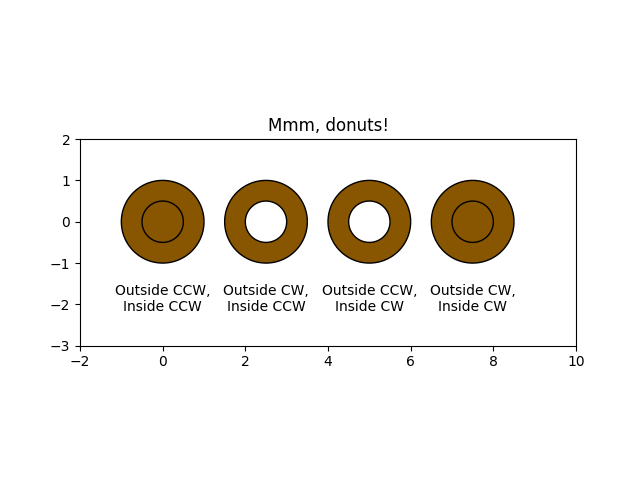# Mmh Donuts!!!#

Draw donuts (miam!) using Paths and PathPatches. This example shows the effect of the path's orientations in a compound path.

import numpy as np
import matplotlib.path as mpath
import matplotlib.patches as mpatches
import matplotlib.pyplot as plt

def wise(v):
if v == 1:
return "CCW"
else:
return "CW"

def make_circle(r):
t = np.arange(0, np.pi * 2.0, 0.01)
t = t.reshape((len(t), 1))
x = r * np.cos(t)
y = r * np.sin(t)
return np.hstack((x, y))

Path = mpath.Path

fig, ax = plt.subplots()

inside_vertices = make_circle(0.5)
outside_vertices = make_circle(1.0)
codes = np.ones(
len(inside_vertices), dtype=mpath.Path.code_type) * mpath.Path.LINETO
codes = mpath.Path.MOVETO

for i, (inside, outside) in enumerate(((1, 1), (1, -1), (-1, 1), (-1, -1))):
# Concatenate the inside and outside subpaths together, changing their
# order as needed
vertices = np.concatenate((outside_vertices[::outside],
inside_vertices[::inside]))
# Shift the path
vertices[:, 0] += i * 2.5
# The codes will be all "LINETO" commands, except for "MOVETO"s at the
# beginning of each subpath
all_codes = np.concatenate((codes, codes))
# Create the Path object
path = mpath.Path(vertices, all_codes)
patch = mpatches.PathPatch(path, facecolor='#885500', edgecolor='black')

ax.annotate("Outside %s,\nInside %s" % (wise(outside), wise(inside)),
(i * 2.5, -1.5), va="top", ha="center")

ax.set_xlim(-2, 10)
ax.set_ylim(-3, 2)
ax.set_title('Mmm, donuts!')
ax.set_aspect(1.0)
plt.show()References

The use of the following functions, methods, classes and modules is shown in this example:

Keywords: matplotlib code example, codex, python plot, pyplot Gallery generated by Sphinx-Gallery